# Tensor product

(diff) ← Older revision | Latest revision (diff) | Newer revision → (diff)
Jump to: navigation, search

The tensor product of two unitary modulesand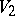over an associative commutative ringwith a unit is the-module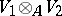together with an-bilinear mapping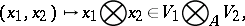which is universal in the following sense: For any-bilinear mapping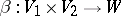, whereis an arbitrary-module, there is a unique-linear mapping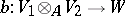such that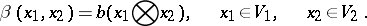The tensor product is uniquely defined up to a natural isomorphism. It always exists and can be constructed as the quotient module of the free-modulegenerated by the set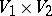modulo the-submodulegenerated by the elements of the form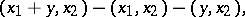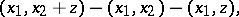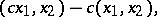then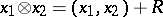. If one gives up the requirement of commutativity of, a construction close to the one described above allows one to form from a right-moduleand a left-modulean Abelian group, also called the tensor product of these modules . In what followswill be assumed to be commutative.

The tensor product has the following properties: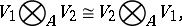for any-modules,and.

If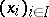and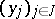are bases of the free-modules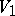and, thenis a basis of the module. In particular,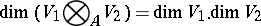if theare free finitely-generated modules (for instance, finite-dimensional vector spaces over a field). The tensor product of cyclic-modules is computed by the formulawhereand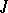are ideals in.

One also defines the tensor product of arbitrary (not necessarily finite) families of-modules. The tensor productis called the-th tensor power of the-module; its elements are the contravariant tensors (cf. Tensor on a vector space) of degreeon.

To any pair of homomorphisms of-modules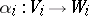,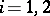, corresponds their tensor product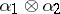, which is a homomorphism of-modulesand is defined by the formula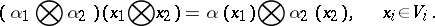This operation can also be extended to arbitrary families of homomorphisms and has functorial properties (see Module). It defines a homomorphism of-modules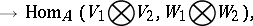which is an isomorphism if all the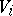andare free and finitely generated.

## Contents

How to Cite This Entry:
Tensor product. Encyclopedia of Mathematics. URL: http://encyclopediaofmath.org/index.php?title=Tensor_product&oldid=12437
This article was adapted from an original article by A.L. Onishchik (originator), which appeared in Encyclopedia of Mathematics - ISBN 1402006098. See original article